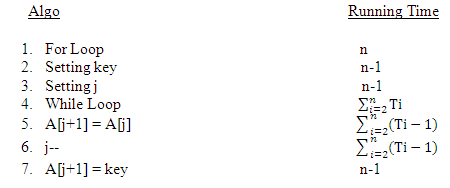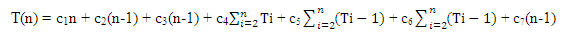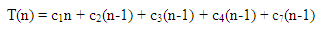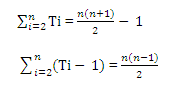# Implementation of Insertion Sort with C# and analysis of running time on best, worst and average case

 Category: Data Structure And Algorithms Tags:Insertion Sort Code Files

## Introduction

When we study data structure and algorithms, sorting algorithms are vital. One of the common sorting algorithm is Insertion Sort, which is similar how we sort deck of cards in our hands.Insertion sort is nothing but inserting elements at their right position comparing others. To implement this algorithm we are always given an array of unsorted elements and using the algorithm we can sort the array.

## Implementation

We will use C# for the Implementation but at the level of data structure and algorithms we can use any programming language it's all about choosing right algorithm and efficiency of an algorithm.

```public class Insertion
{
int[] A;   //Array which has to sort
public Insertion(int[] array) //Constructor
{
A = array;
}
/// <summary>
/// Sorts in Increasing order
/// </summary>
/// <returns></returns>
public int[] SortIncr()
{
for (int i = 1; i < A.Length; i++)
{
int key = A[i];
int j = i - 1;
/* below while loop traverse all the elements which are before the
key element from right to left or breaks if finds a small element
than key */
while (j > -1 && A[j] > key)
{
A[j + 1] = A[j]; // moving the element to next position
j--;
}
A[j + 1] = key; // **Imp, It is inserting key element on right position
}
return A;
}
/// <summary>
/// Sorts in decreasing order
/// </summary>
/// <returns></returns>
public int[] SortDecr()
{
for (int i = 1; i < A.Length; i++)
{
int key = A[i];
int j = i - 1;
while (j > -1 && A[j] < key)
{
A[j + 1] = A[j];
j--;
}
A[j + 1] = key;
}
return A;
}
/// <summary>
/// Prints all the elements of the array
/// </summary>
public void Print()
{
if (A != null)
{
foreach (int i in A)
{
Console.WriteLine(i);
}
}
}
}
```

The above example is self explanatory with comments. I will explain SortIncr method:

1. The loop runs from 2nd element of array to last, no need to start from 1st element because no element is there before 1st element to compare.
2. Setting key element to compare.
3. Initializing j with the just previous index of key element.
4. The variable j loops until first element or it finds bigger element than key, replace all the elements before key elements to immediate next location and insert key on its right position.Image Reference: Wikipedia

Let's run above code:

`Insertion insertionSort = new Insertion(new int[] { 2, 1, 7, 4, 9, 3, 6 });var sortedArr = insertionSort.SortIncr();foreach (int ele in sortedArr)    Console.WriteLine(ele);Console.ReadLine();`

Output:

1

2

3

4

6

7

9

## Analysis

Analysis of any algorithm is necessary which comes up with the efficiency of an algorithm. Below is the running time of each step:Here let’s suppose we are having an array of size n so the for-loop will run from 2nd element to n'th and running time of for-loop will be n(because control hit for-loop n+1 times for going 1 to n). Running time should be n+1 but we are starting from 2nd element so it is n.

In step 5: when key is 3rd element of array, step 5 will execute 2 times (to compare 2nd and 1st element if it is worst case)
So the running time:Best Case: When array is already sorted, the step 5 and 6 will never run,That is only linear function of n, so in best case time complexity will be O(n).

Worst Case: When array is sorted in reverse mannerSo, when we place the value of these summations in above algorithm, we find the n(n-1) factor that is (n2 - n) so we can say the complexity will rely on the factor n2 so that complexity in worst case will be O(n2) .

Average Case: The average case of any algorithm will be near to worst case in this case if we replace Ti section by Ti/2 for average, things in sights of complexity will be same and the time complexity will be same as worst case.

 Like0 People
Last modified on 29 October 2018Nikhil Joshi Ceo & Founder at Dotnetlovers Atricles: 132 Questions: 9 Given Best Solutions: 9 *

## Comments:

No Comments Yet

You are not loggedin, please login or signup to add comments:

## Existing User

Login via:## New Userx